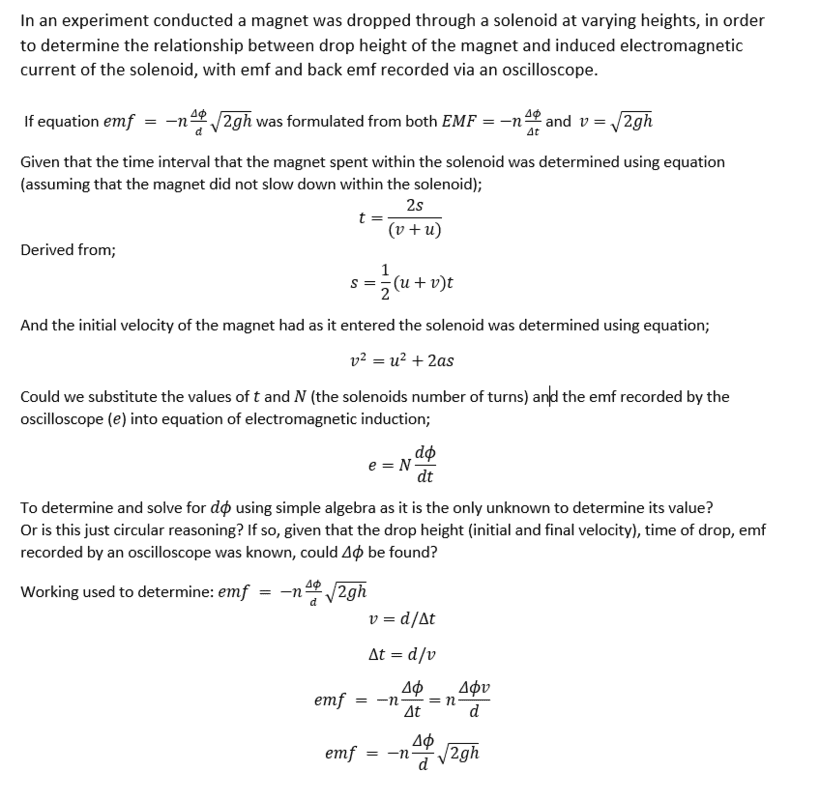# Electromagnetic induction (finding ΔΦ).

KarlsShwarzschildGold Member
2022 Award

I am unsure if I understand what you are doing. It seems ##\frac{d}{\sqrt{2gh}}## is approximately the time that the magnet spends inside the coil. You are also using $$\mathcal{E} = -N\frac{d\Phi}{dt}$$I suppose the average rate of change of flux in a time ##\Delta t## is ##\frac{\Delta \Phi}{\Delta t}##, from which it follows that the average EMF ##\mathcal{E} = -N \frac{\Delta \Phi}{\Delta t}##. So your construction ##\mathcal E = -N \frac{\Delta \Phi}{d}\sqrt{2gh}## is the time-averaged EMF inside the coil whilst the magnet is inside the coil. However this is zero, because ##\frac{1}{T} \int_0^T -N\frac{d\Phi}{dt} dt = 0##, since the flux linked in the coil is the same when the magnet is at the top of the coil as when it leaves (I assume necessary symmetry here).

Plus, the magnet actually still accelerates inside the coil, so a graph of ##\mathcal{E}## vs ##t## has an uneven shape (but still a total integral of 0).

•berkeman
Mentor
I'm not too sure if I overestimated the prefix, guess we'll find out••rude man and berkeman